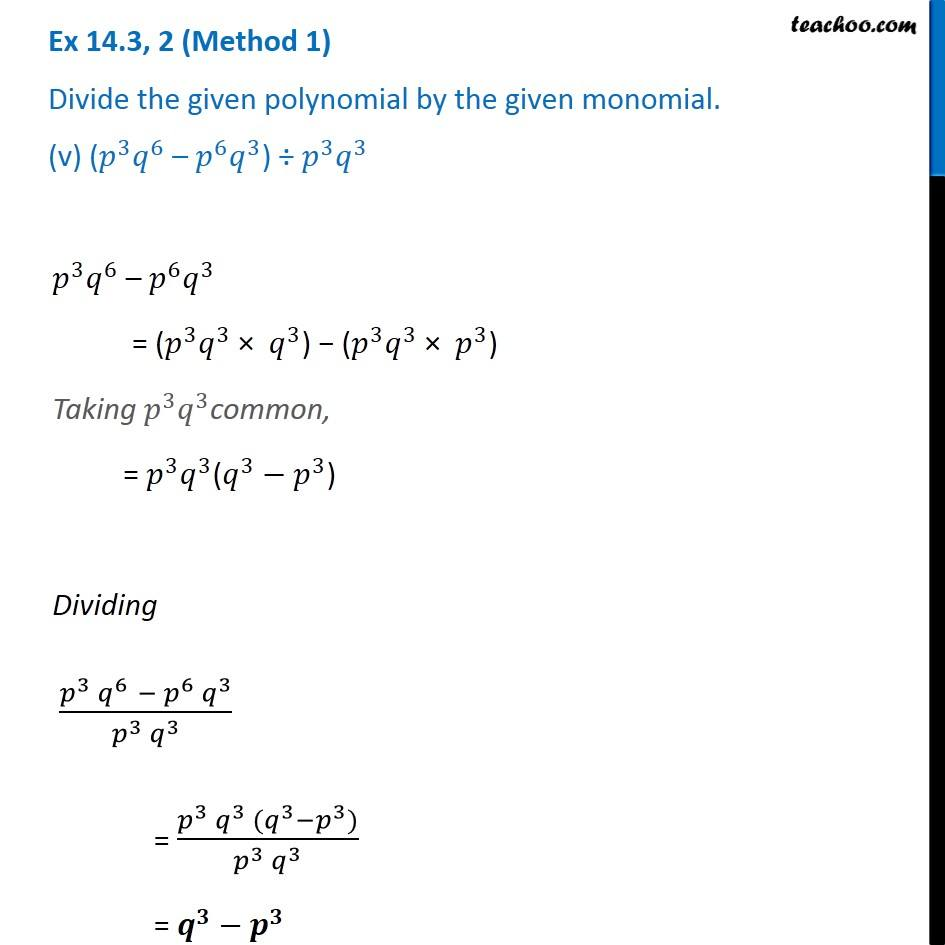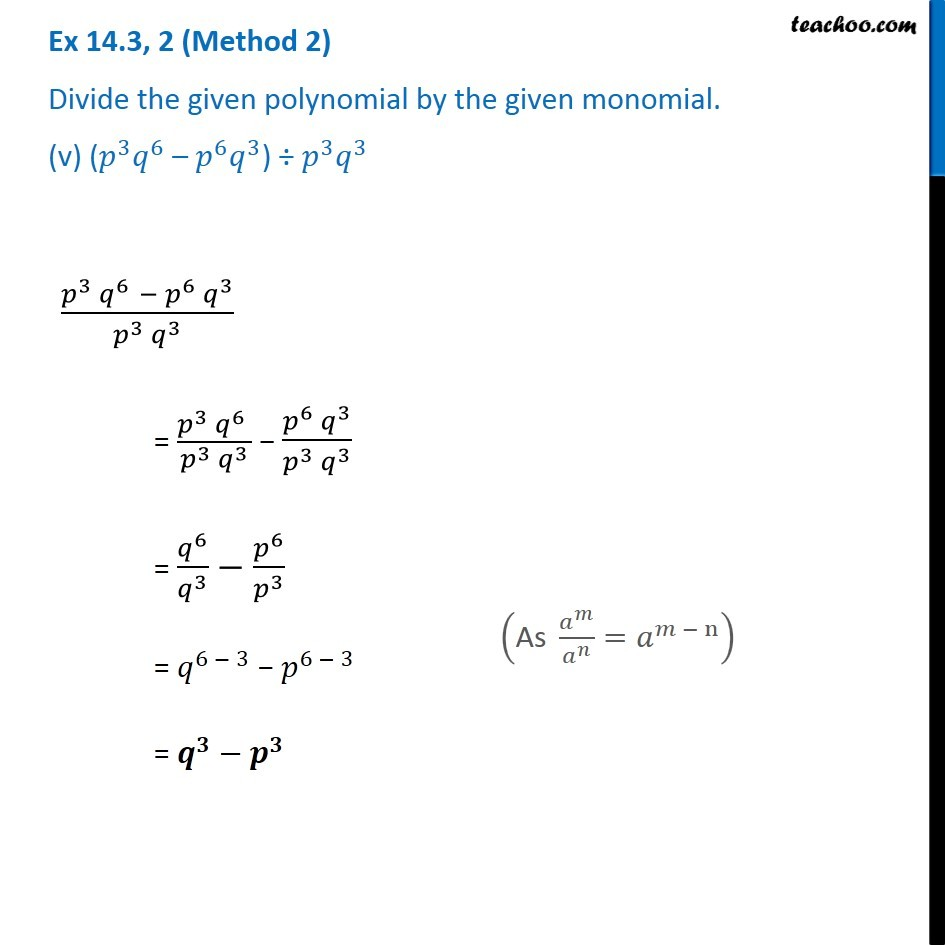Dividing polynomial by monomial

Chapter 12 Class 8 Factorisation
Concept wiseLearn in your speed, with individual attention - Teachoo Maths 1-on-1 Class

### Transcript

Ex 12.3, 2 (Method 1) Divide the given polynomial by the given monomial. (v) (𝑝^3 𝑞^6 – 𝑝^6 𝑞^3) ÷ 𝑝^3 𝑞^3 𝑝^3 𝑞^6 – 𝑝^6 𝑞^3 = (𝑝^3 𝑞^3 × 𝑞^3) − (𝑝^3 𝑞^3 × 𝑝^3) Taking 𝑝^3 𝑞^3common, = 𝑝^3 𝑞^3(𝑞^3−𝑝^3) Dividing (𝑝^3 𝑞^6 " " −〖 𝑝〗^6 𝑞^3)/(𝑝^3 𝑞^3 ) = (𝑝^3 𝑞^3 (𝑞^3−𝑝^3))/(𝑝^3 𝑞^3 ) = 𝒒^𝟑−𝒑^𝟑 Ex 12.3, 2 (Method 2) Divide the given polynomial by the given monomial. (v) (𝑝^3 𝑞^6 – 𝑝^6 𝑞^3) ÷ 𝑝^3 𝑞^3 (𝑝^3 𝑞^6 " " −〖 𝑝〗^6 𝑞^3)/(𝑝^3 𝑞^3 ) = (𝑝^3 𝑞^6 )/(𝑝^3 𝑞^3 ) − (𝑝^6 𝑞^3)/(𝑝^3 𝑞^3 ) = 𝑞^6/𝑞^3 −𝑝^6/𝑝^3 = 𝑞^(6 − 3) − 𝑝^(6 − 3) = 𝒒^𝟑−𝒑^𝟑 ("As" 𝑎^𝑚/𝑎^𝑛 =𝑎^(𝑚 − n) )